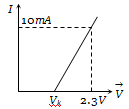The resistance of a germanium junction diode whose is shown in figure is $\left({\mathrm{V}}_{\mathrm{k}}=0.3\mathrm{V}\right)$(a) 5 k$\mathrm{\Omega }$
(b) 0.2 k$\mathrm{\Omega }$
(c) 2.3 k$\mathrm{\Omega }$
(d) $\left(\frac{10}{2.3}\right)\mathrm{k\Omega }$